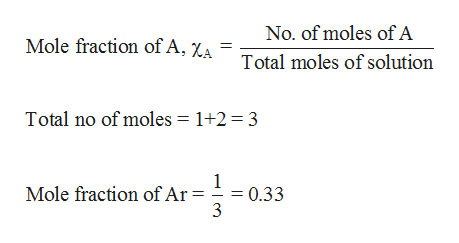# A mixture of Ne and Ar gases at a PT of 1.4atm. If there are twice as many mols of Ne as Ar, what is the partial pressure of Ar (PAr).

Question

A mixture of Ne and Ar gases at a PT of 1.4atm. If there are twice as many mols of Ne as
Ar, what is the partial pressure of Ar (PAr).

check_circleExpert Solution
Step 1

The mixture contains Ne and Ar in the ratio 2:1. Henc...help_outlineImage TranscriptioncloseNo. of moles of A Mole fraction of A, Za Total moles of solution Total no of moles 1+2 = 3 1 0.33 3 Mole fraction of Ar = fullscreen

### Want to see the full answer?

See Solution

#### Want to see this answer and more?

Solutions are written by subject experts who are available 24/7. Questions are typically answered within 1 hour*

See Solution
*Response times may vary by subject and question
Tagged in

### Gas laws